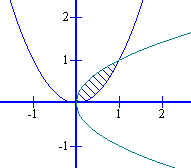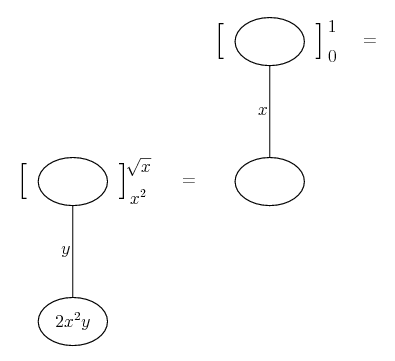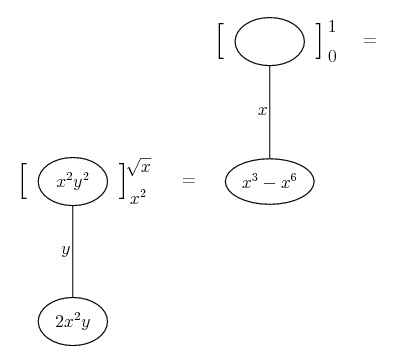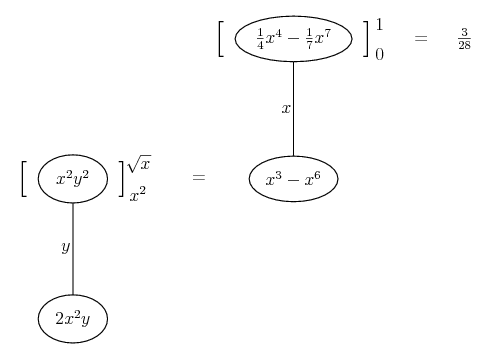# Thread: Volume Integral

1. ## Volume Integral

Let R be the closed region in the xy-plane bounded by the graphs of $\displaystyle y = x^2$ and $\displaystyle x = y^2$. Let V be the solid bounded above by the surface $\displaystyle z = 2x^2y$ whose base is the region R. Calculate the volume of V.

2.So the integral for R would be

$\displaystyle \displaystyle \int _0^1 (\sqrt{x} - x^2)\ dx$

... so the volume integral is

$\displaystyle \displaystyle \int _0^1 \int_{x^2}^{\sqrt{x}} f(x,y)\ dy\ dx$

or...Spoiler:Spoiler:__________________________________________

Don't integrate - balloontegrate!

Balloon Calculus; standard integrals, derivatives and methods

Balloon Calculus Drawing with LaTeX and Asymptote!

#### Search Tags

integral, volume